# Problem 56353. Convert Volume Mixing Ratio to Density

The partial pressure of a constituent gas species of the atmosphere can be expressed in parts per million by volume. For example, the amount of carbon dioxide in the atmosphere is about 400 ppm.
Write a script that takes as input parameters the air parcel characteristics at some altitude above ground:
• Observed Temperature of Air in K
• Observed Pressure of Air in mb
• The (string) name of the gas consituent
• The parts per million (by volume) of the gas consituent
and outputs:
• The density of the gas consituent (in mg/m^3)
If the input gas name is 'DRY_AIR' and the partial pressure in PPMV is 1e6 (all the particles), the output will be the mass density of the air (roughly, since there are slight changes for humidity). For other molecules, such as CO2 (carbon dioxide) or O3 (ozone), the value is the mass density of those consituents of the atmosphere.
To make the conversion, first find the molecular density (molecules/cm3) of air (mix of all gases N2, O2, etc.) for the given temperature and pressure.
The molecular density (also known as the number density) of air can be found from the Temperature and Pressure using the ideal gas law.using Loschmidt’s number STP:
•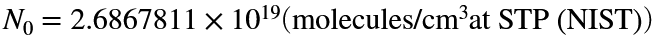And STP Temperature and Pressure:
•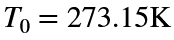•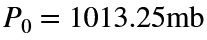Convert the molecular density to the mass density by multiplying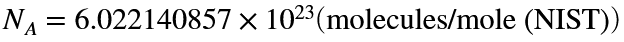and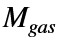=the molar mass (g/mole) of the molecule.
The molar mass of ozone ('O3') is 47.9982. The molar mass of carbon dioxide ('CO2') is 44.010. The function template has a lookup table of common atmosphere gas constituents.

### Solution Stats

24.71% Correct | 75.29% Incorrect
Last Solution submitted on Dec 03, 2023

### Community Treasure Hunt

Find the treasures in MATLAB Central and discover how the community can help you!

Start Hunting!